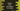# Python program to find all numbers in a string## Introduction :

In this tutorial, we will learn how to find out all numbers in a string using python. The program will take one string as an input from the user. It will find out all numbers in that string and print them on the console.

We will learn two different ways to solve this problem. The first method will iterate through the string and check for each character if it is a number or not and the second method will use one lambda and the third method will use one regular expression or Regex to find out all numbers in one go.

## Approach 1: Iterating through all characters :

In this approach, we will iterate through each character of the string one by one. The complete program will look like as below :

``````str = input("Enter a string : ")

for c in str:
if(c.isdigit()):
print(c)``````

It uses one for loop to iterate through the characters of the string. isdigit() checks if a character is digit or not.

## Approach 2: using lambda :

``````str = input("Enter a string : ")

digits = list(filter(lambda ch: ch.isdigit(), str))

print(digits)``````

The filter method will filter out all digits from the string str and generate one list from these values. digits is the final list.

Sample output :

``````Enter a string : Hello2 w3r1d5 !0
['2', '3', '1', '5', '0']``````

## Approach 3: using a regex :

``````import re

str = input("Enter a string : ")

digits = re.findall(r"\d",str)

print(digits)``````

It will produce output like below :

``````Enter a string : he33llo wo4
['3', '3', '4']``````

\d is the same as [0-9] i.e. it is used to match all numbers.

This example considers all numbers as a single digit, i.e. it treats 33 as two 3s. Replace \d with \d+ if you want all numbers with single or multiple digits.

``````import re

str = input("Enter a string : ")

digits = re.findall(r"\d+",str)

print(digits)``````

For the same above example :

``````Enter a string : he33llo wo4
['33', '4']``````

### Conclusion :

I have listed here a couple of different ways to find all numbers in a python string. Drop a comment below if you know any other ways to solve it. All of these programs are for python 3. Try to go through them and drop one comment below if you have any questions.